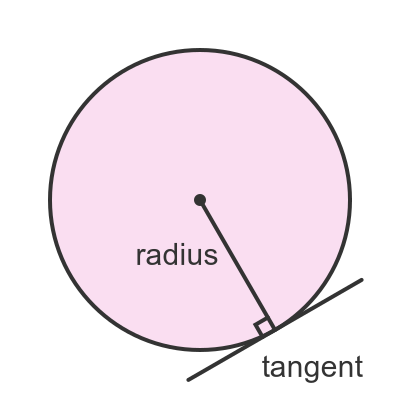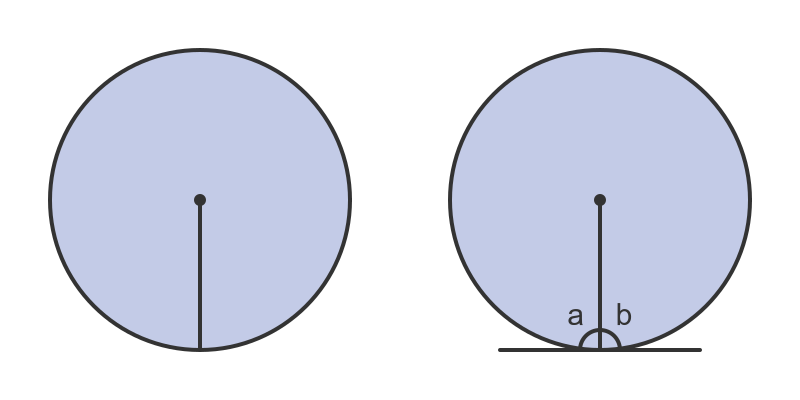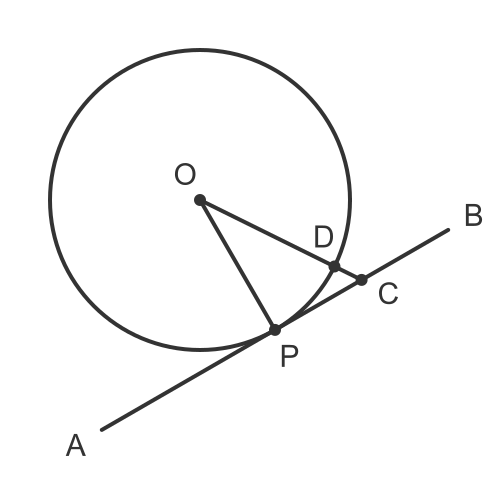# Tangent and radius of a circle meet at 90°

By Martin McBride, 2022-12-06
Categories: gcse geometryA tangent is a line that just touches the circle at a single point on its circumference.If we draw a radius that meets the circumference at the same point, the angle between the radius and the tangent will always be exactly 90°.

This theorem is covered in this video:

## Intuitive argument

We can use symmetry to provide an intuitive argument as to why this might be true.When we draw a radius vertically (circle on the left), the diagram is symmetrical. The left half of the circle is a mirror image of the right half.

If we add a tangent (circle on the right), then the radius meets the tangent forming an angle a on the left and b on the right.

Due to symmetry, there is no reason to assume that either a > b or a > b. Intuitively it seems reasonable to assume that the two angles are equal, and since they add to make a straight line they would each be 90 degrees.

This is not a proof, it is just a way of convincing ourselves that it is probably true. The proof is next.

## Proof

You aren't required to learn this proof for GCSE, it is just here for information.

We want to prove that the angle between the radius OP and the tangent AB is a right angle.

We will use proof by contradiction. This means that we will start by assuming that the statement is not true. This will lead to a contradiction, proving that it is impossible for the statement to be not true, which means it must be true.

So we start with the assumption that the line OP does not meet the line AB at a right angle.

There must be some other line from O that meets the line AB at a right angle. It meets the line AB at some point C. Here is a diagram:Since the circle only touches the tangent in one place, point P, it follows that the line OC must cross the circle at some point. We will call that point D.

We have deliberately chosen point C such that the angle ∠OCA is a right angle. So we can now draw just the triangle on its own:One thing to notice here is that the lines OD and OP are both radii of the circle, so they both have equal lengths. Since the line OC clearly longer than OD, it must also be longer than OP

But this is an impossible triangle, because:

• Angle C is the right angle, so side OP must be the hypotenuse.
• The hypotenuse, OP of a triangle is always the longest side.
• But we know that OC is always longer than OP.

This is a contradiction. It means that it is impossible for line OC to meet line AB at a right angle.

Now C can be placed anywhere on the line AB except at position P. But where ever we place it, the angle ∠ACB cannot be a right angle.

There must be a line from O that meets AB at a right angle, and it can't be at any position C that isn't P, so it must be at position P.

OP is the radius, and AB is the tangent, and we have proved that they meet at a right angle.